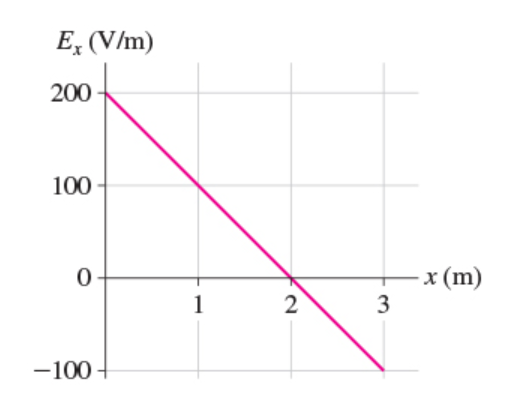# Problem: What is the potential difference between xi = 2.0 m and xf = 3.0 m ? Express your answer to two significant figures and include the appropriate units.

###### FREE Expert Solution

Potential difference:

$\overline{){\mathbf{∆}}{\mathbf{V}}{\mathbf{=}}{\mathbf{-}}{\mathbf{\int }}{\mathbf{E}}{\mathbf{\left(}}{\mathbf{x}}{\mathbf{\right)}}{\mathbf{d}}{\mathbf{x}}}$

Potential difference is the negative area under the curve.

79% (205 ratings)###### Problem Details

What is the potential difference between xi = 2.0 m and xf = 3.0 m ? Express your answer to two significant figures and include the appropriate units.